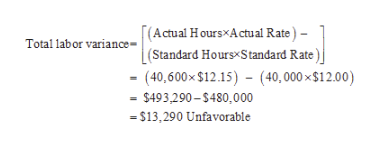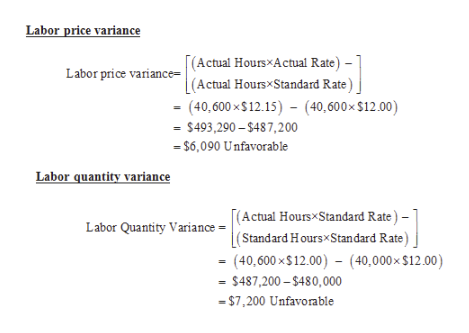# Adams Company’s standard labor cost of producing one unit of Product DD is 4.00 hours at the rate of \$12.00 per hour. During August, 40,600 hours of labor are incurred at a cost of \$12.15 per hour to produce 10,000 units of Product DD.a) What is the total labor variance?b) What is the labor price and quantity variances?c) What is the labor price and quantity variances, assuming the standard is 4.10 hours of direct labor at \$12.25 per hour?

Question
56 views

Adams Company’s standard labor cost of producing one unit of Product DD is 4.00 hours at the rate of \$12.00 per hour. During August, 40,600 hours of labor are incurred at a cost of \$12.15 per hour to produce 10,000 units of Product DD.

a) What is the total labor variance?

b) What is the labor price and quantity variances?

c) What is the labor price and quantity variances, assuming the standard is 4.10 hours of direct labor at \$12.25 per hour?

check_circle

Step 1

(a) Compute direct labor variance.help_outlineImage Transcriptionclose(Actual HoursxActual Rate)- |(Standard Hours Standard Rate ) (40,600x \$12.15) (40,000x\$12.00) Total labor variance \$493,290-\$480,000 -\$13,290 Unfavorable fullscreen
Step 2

(b) Compute labor price variance and labor...help_outlineImage TranscriptioncloseLabor price variance [(Actual HoursxActual Rate) -- (Actual HoursxStandard Rate) (40,600x\$12.15) (40,600x \$12.00) Labor price variance= \$493,290-\$487,200 -\$6,090 Unfavorable Labor quantity variance Labor Quantity Variance=Actual Hours Standard Rate) - (Standard Hours Standard Rate) - (40,600 x\$12.00) - (40,000x \$12.00) = \$487,200-\$480,000 \$7,200 Unfavorable fullscreen

### Want to see the full answer?

See Solution

#### Want to see this answer and more?

Solutions are written by subject experts who are available 24/7. Questions are typically answered within 1 hour.*

See Solution
*Response times may vary by subject and question.
Tagged in